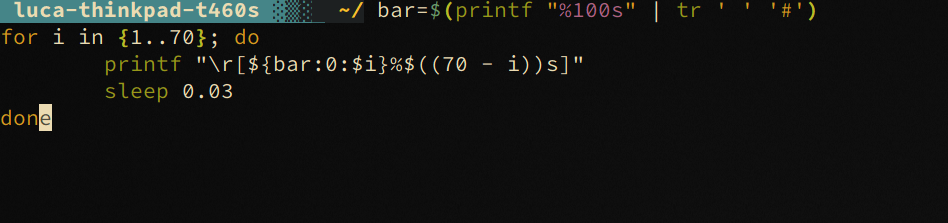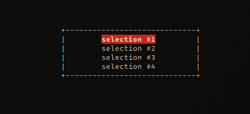\$ cyanpencil's blog - posts - about - contact

# Cool bash stuff with ANSI escape codes

Let’s see a few fun stuff you can do with a bit of bash and ANSI escape codes

## Example #1: ASCII Progress bar

You know a script is cool when it shows a progress bar. And you write only cool scripts, right?

For this, we don’t need fancy stuff, just the `\r` char will do the magic for us. Let’s also assume you have a `percentage` variable lying around with display the progress of whatever unoptimized thing you’re doing as an integer from 0 to 100.

Python is our friend, and lets us roll with this one-liner:

``````print('\r[' + '#'*percentage + ' '*(100 - percentage) + ']', end=' ')
``````

Pretty straightforward, right? But I hear you, you’re not using python, you’re making a script, so take this bash two-liner:

``````\$ bar=\$(printf "%100s" | tr ' ' '#')
\$ printf "\r[\${bar:0:\$percentage}%\$((100 - percentage))s]"
``````

Ok so there’s some bash fancy stuff. Let’s decompose that a bit:

• `\$(printf "%100s" | tr ' ' '#')` - makes a string with 100 spaces, and replaces them with `#`
• `\${bar:0:\$percentage}` bash’s magic variable substitution, works like python’s `bar[0:percentage]`
• `%\$((100 - percentage))s` printing the remaining spaces until we get to a length of 100

For specimen demonstration purposes, I stuck that in a loop for you to show it off:

``````bar=\$(printf "%100s" | tr ' ' '#')
for i in {1..100}; do
printf "\r[\${bar:0:\$i}%\$((100 - i))s]"
sleep 0.03
done
``````

Results:## Example #2: bash selection menu

Using the escape sequence`\x1b[y;xH` we can move the cursor to the `(x,y)` coordinates in the terminal. We also know that `\e[?25l` hides the cursor and `\e[?25h` shows it back.

``````#!/bin/bash

# get terminal size
read LINES COLUMNS < <(stty size)

# available selections (colon separated)
IFS=: read -a text <<< "selection #1:selection #2:selection #3:selection #4"
w=30
h=\$((\${#text[@]}+1))
x=\$((COLUMNS/2 - w/2))
y=\$((LINES/2 - h/2))

sel=0

# hide cursor
printf "\e[?25l"

# line (bool vert, int x, int y, int len)
function line () {
if [[ \$1 == v ]]; then
for (( i = 1; i < \$4; i++)); do
printf "\x1b[\$((\$3 + \$i));\$((\$2))H|"
done
else
for (( i = 1; i < \$4; i++)); do
printf "\x1b[\$((\$3));\$((\$2 + \$i))H-"
done
fi
}

while true; do
# erase screen
printf "\x1b[2J"

# box borders
line h \$x       \$y       \$w
line h \$x       \$((y+h)) \$w
line v \$x       \$y       \$h
line v \$((x+w)) \$y       \$h

# box corners
printf "\x1b[\$((y+h));\$((x+w))H+"
printf "\x1b[\$((y));\$((x+w))H+"
printf "\x1b[\$((y+h));\$((x))H+"
printf "\x1b[\$((y));\$((x))H+"

# display text selections
for ((i = 0; i < \${#text[@]}; i++)); do
printf "\x1b[\$((y+i+1));\$((x+w/2-\${#text[i]}/2))H"
if [[ \$sel -eq \$i ]]; then
printf "\x1b[41;1m\${text[i]}\x1b[0m"
else
printf "\${text[i]}"
fi
done

# read only one char of input
case \$input in
j)  sel=\$(((sel+1) % \${#text[@]})) ;;
k)  sel=\$(((sel-1) % \${#text[@]}))
if [[ sel -lt 0 ]]; then sel=3; fi ;;
"") printf "\x1b[\$((y+h+1));0H"
echo "You selected \${text[\$sel]}"
break ;;
q)  break ;;
esac
done

# show cursor when we're done
printf "\e[?25h"
``````

This is the result, moving the selection up and down with `j` and `k` keys: